IntroductionIntroduction# Surface Area of Cones

INTRO
The surface area of a 3D shape is equal to the sum of the areas of all the faces (sides) of that shape. A helpful way to think about it is:
surface area = sum of face areas
We can also think about surface area in terms of gifts! The surface area is like the minimum amount of wrapping paper we need to prep a gift for its surprise reveal. 🤩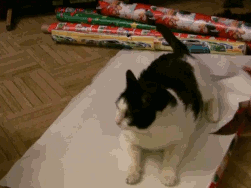If we were to unwrap a gift, the amount of wrapping would be equal to the surface area of the object it was covering.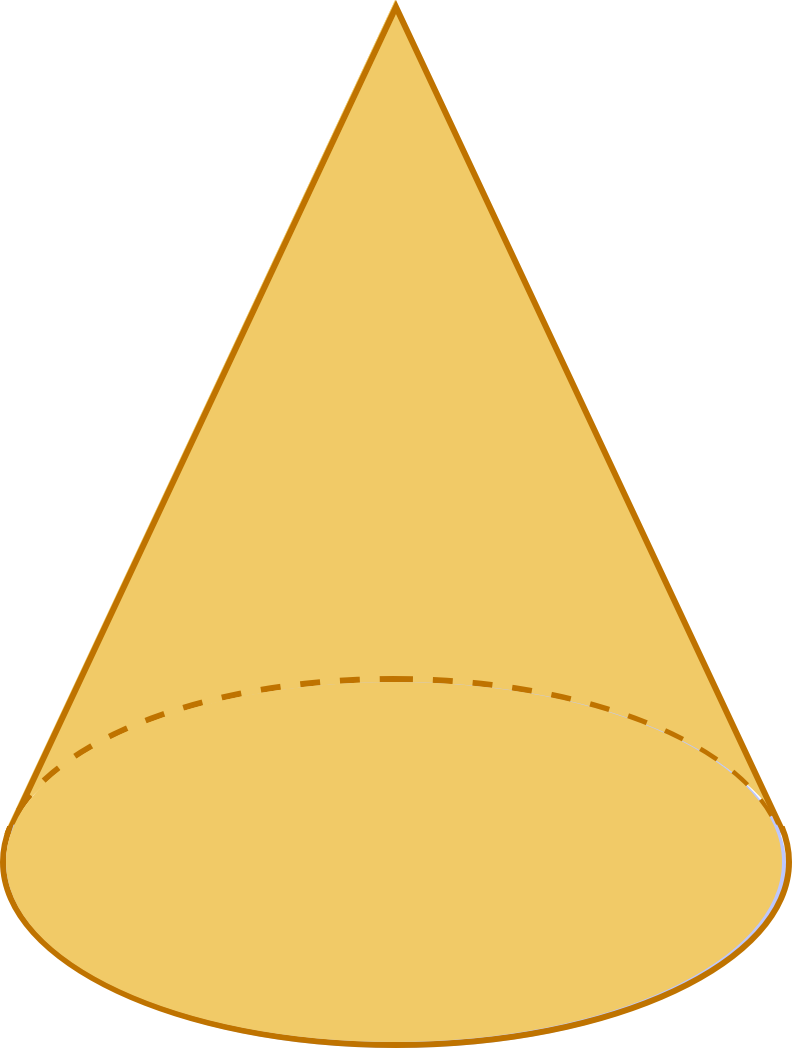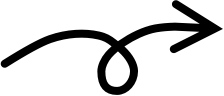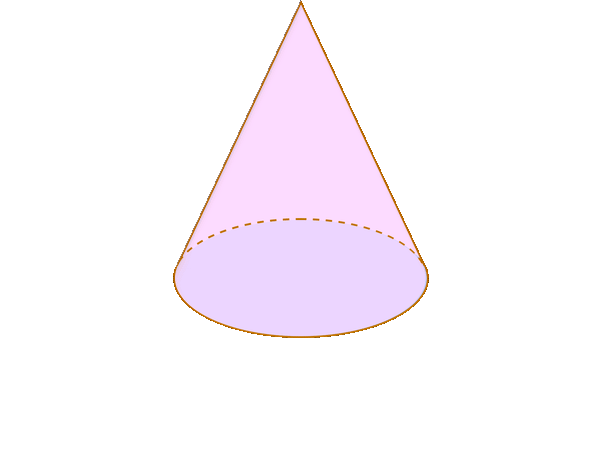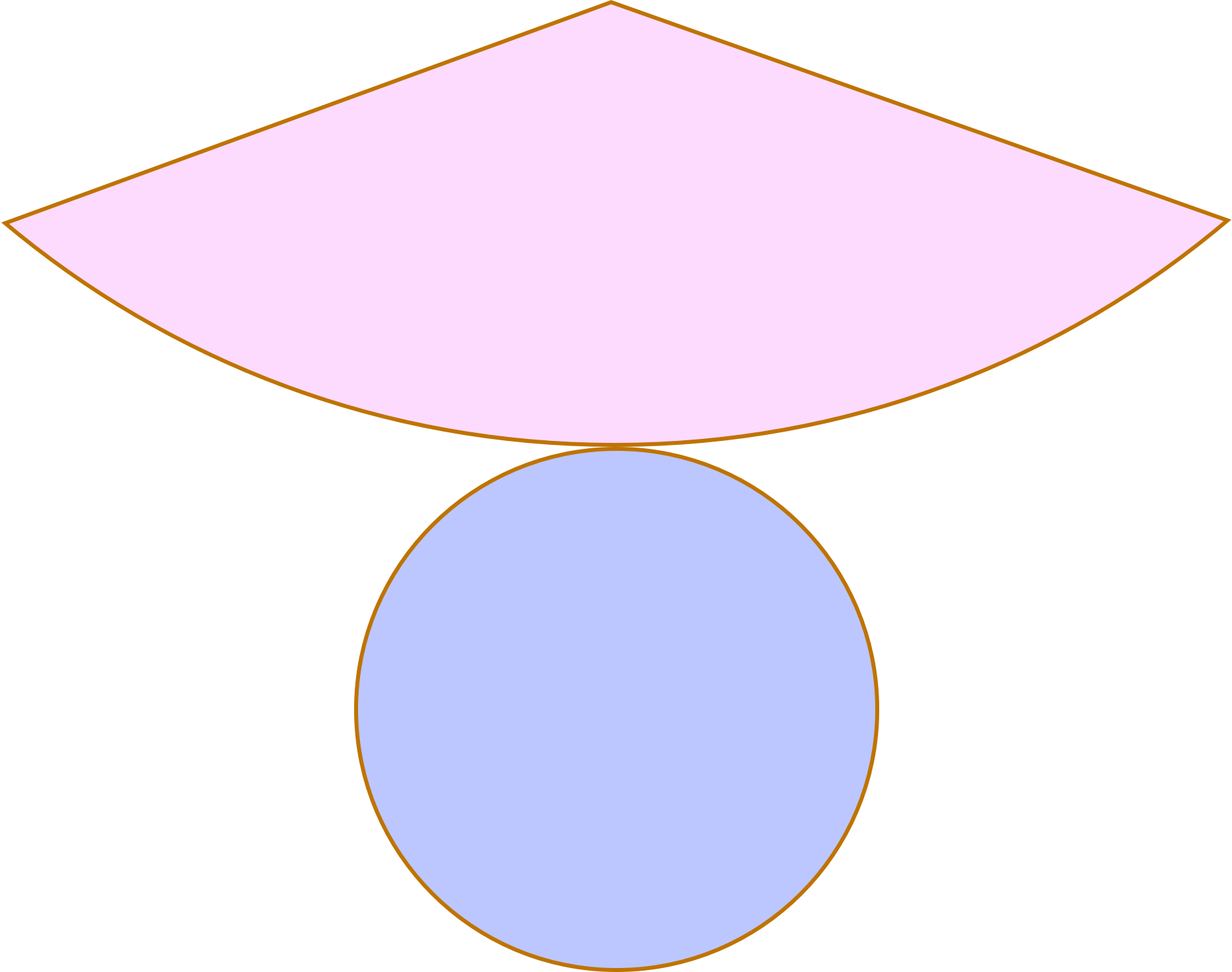When we “unwrap” a 3D shape, we get what we call a net. A surface area net shows us the different 2D shapes that make up the faces of the 3D shape.
We can then find the area of each face and then add them all together to find the total surface area.

### What is lateral surface area?

When working with pyramids, cylinders, and cones, you may also be asked to find the lateral surface area.
Lateral surface area is simply the total surface area of an object minus the area of its base(s).
Check out our
Calculator
or explore our
Lesson
and
Practice

CALCULATOR

## Surface Area of Cones Calculator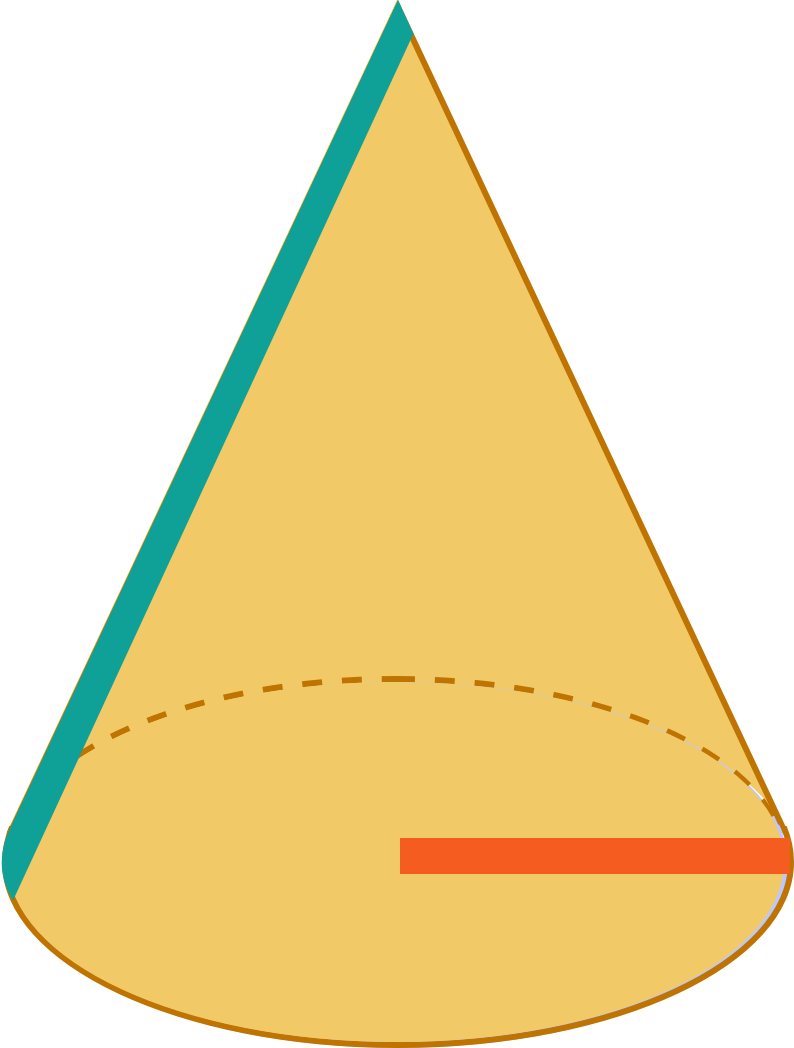KEY STEPS

## How to Find Surface Area of Cones

### Step 1. Unwrap the cone.### Step 2. Calculate the area of each part of the net that makes up the cone.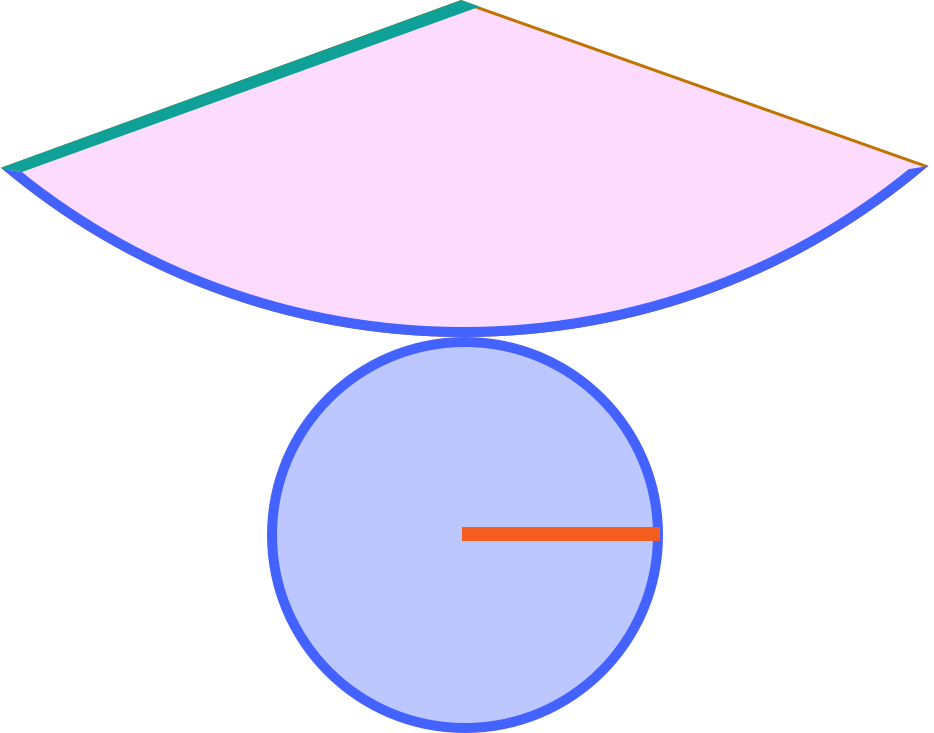Sector Circle

### Step 3. Add up the areas of the shapes that make up the cone.

LESSON
Surface Area of Cones

## Surface Area of Cones

The surface area of a cone is equal to the sum of the area of the rolled side and area of the base of the cone.
If we unwrap a cone, we can see that the net is made up of circle and portion of a larger circle, which we call a sector.So, we need to find the area of the circle () and the area of the sector (), and then add them together.
PRACTICE
Surface Area of Cones

## Practice: Surface Area of Cones

Question 1 of 5: Find the surface area of this cone:### Step 1. Unwrap the cone.CONCLUSION
Nice work, look at you go! Thanks for checking out this lesson ☺️🙏. Where to next?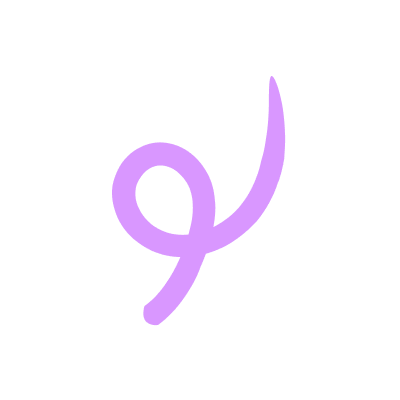Leave Feedback